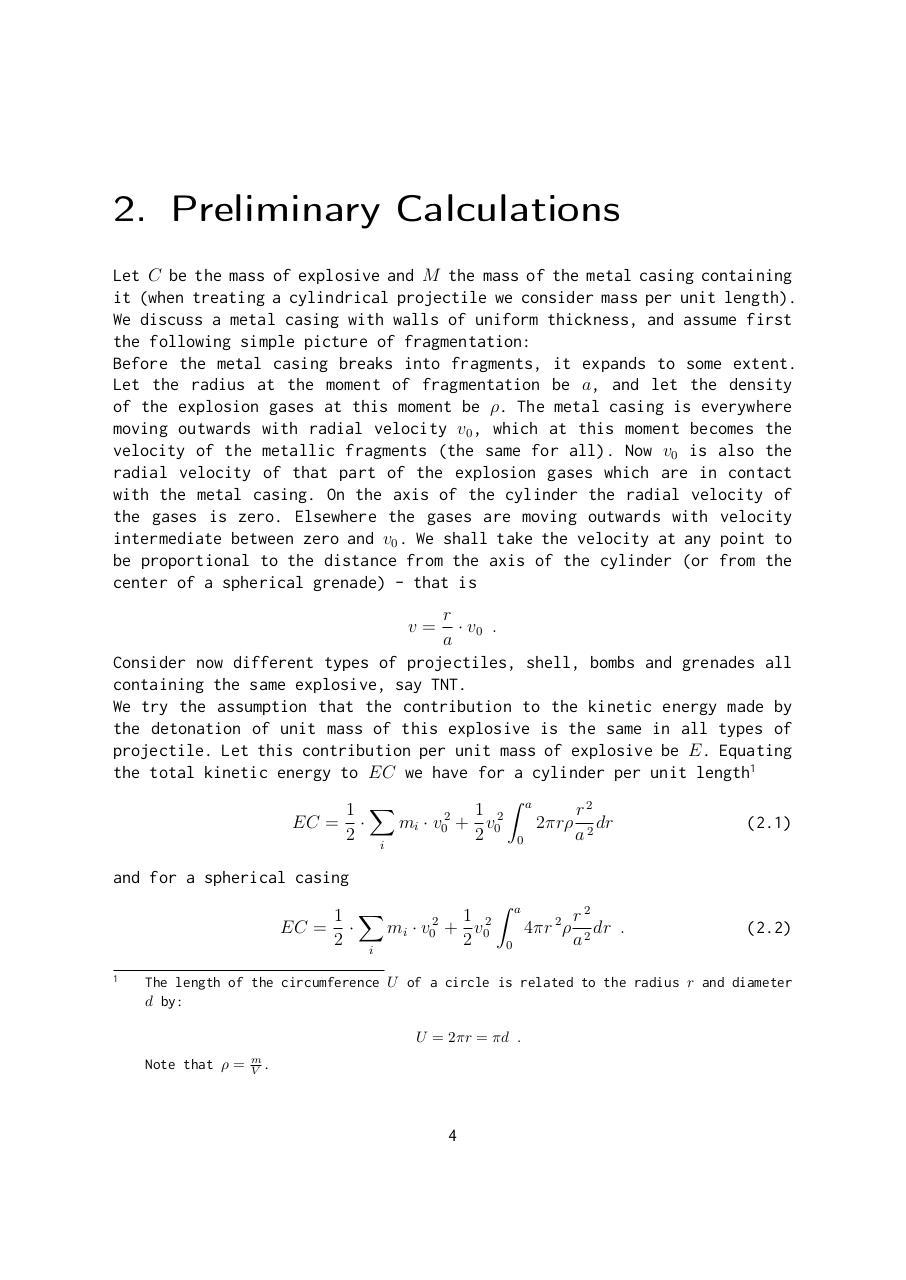# Ronald W. Gurney. The Initial Velocities of Fragments from Bombs, Shell, Grenades.pdfPage 1 2 3 45620

#### Text preview

2. Preliminary Calculations
Let C be the mass of explosive and M the mass of the metal casing containing
it (when treating a cylindrical projectile we consider mass per unit length).
We discuss a metal casing with walls of uniform thickness, and assume first
the following simple picture of fragmentation:
Before the metal casing breaks into fragments, it expands to some extent.
Let the radius at the moment of fragmentation be a, and let the density
of the explosion gases at this moment be ρ. The metal casing is everywhere
moving outwards with radial velocity v0 , which at this moment becomes the
velocity of the metallic fragments (the same for all). Now v0 is also the
radial velocity of that part of the explosion gases which are in contact
with the metal casing. On the axis of the cylinder the radial velocity of
the gases is zero. Elsewhere the gases are moving outwards with velocity
intermediate between zero and v0 . We shall take the velocity at any point to
be proportional to the distance from the axis of the cylinder (or from the
center of a spherical grenade) - that is
r
· v0 .
a
Consider now different types of projectiles, shell, bombs and grenades all
containing the same explosive, say TNT.
We try the assumption that the contribution to the kinetic energy made by
the detonation of unit mass of this explosive is the same in all types of
projectile. Let this contribution per unit mass of explosive be E. Equating
the total kinetic energy to EC we have for a cylinder per unit length1
Z
r2
1 2 a
1 X
2
2πrρ 2 dr
mi · v0 + v0
(2.1)
EC = ·
2 i
2
a
0
v=

and for a spherical casing
Z
r2
1 X
1 2 a
2
4πr 2 ρ 2 dr .
mi · v0 + v0
EC = ·
2 i
2
a
0
1

(2.2)

The length of the circumference U of a circle is related to the radius r and diameter
d by:
U = 2πr = πd .
Note that ρ =

m
V .

4